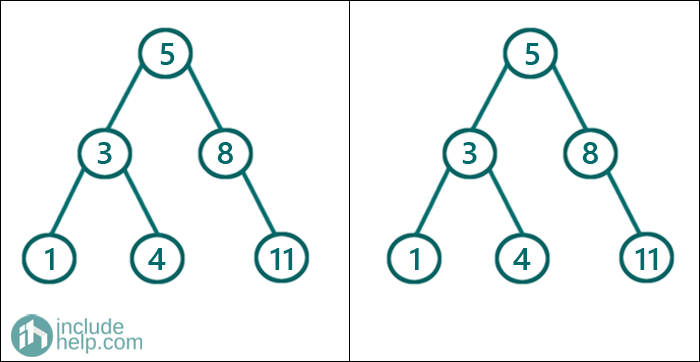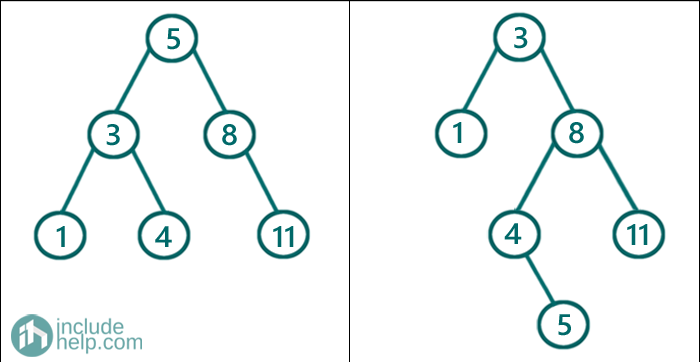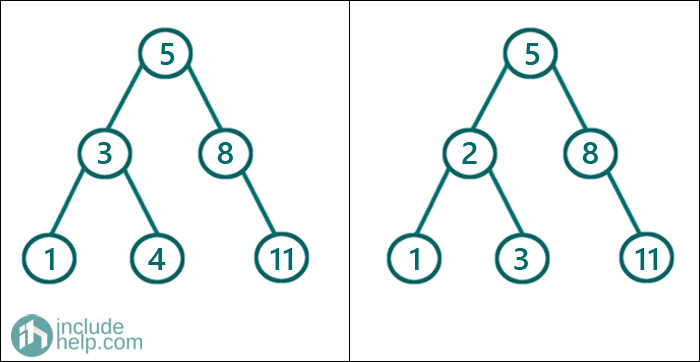# Check if two BSTs have same set of elements or not

In this article, we are going to see how to find whether two BSTs contains same set of elements or not?
Submitted by Radib Kar, on November 01, 2020

In this article, we are going to see whether two BSTs have same set of elements or not. Below are some examples, where we show different cases,

1) Two same BSTsIn the above example, since two BSTs are exactly same, thus they have to have same set of elements.

2) Two different BST but having same set of elementsIn the above example, though two BSTs are not exactly same, they have a same set of elements which is [1, 3, 4, 5, 8, 11]

3) Two BSTs not having same set of elementsThe above BSTs don't have same set of elements as the first one has the set [1, 3, 4, 5, 8, 11] and the second has set [1, 2, 3, 5, 8, 11]

So, what we can do is to do an inorder traversal for the first tree and then we can check whether the inorder traversal of the second tree is same as the first one or not.

So, to do that store the inorder traversal of the first tree. That would be a sorted list. Then do an inorder traversal to check whether the stored sequence is same as the inorder traversal or not. To check that, we do similarly as we did in our last article check given sorted subsequence exist in the BST or not.

The only modification is that here the sorted list needs to be exactly same as the BST inorder traversal. So, we also keep a count which will keep track of how many nodes is there in the 2nd BST. At the end we need to check that both the index has reached end of the array and also the count is same as the size of the stored traversal. If both of this condition becomes true, then we can infer that both the BSTs have same set of elements. Otherwise, they don't have.

Below is the implementation.

```#include <bits/stdc++.h>
using namespace std;

// tree node is defined
class TreeNode {
public:
int val;
TreeNode* left;
TreeNode* right;
TreeNode(int data)
{
val = data;
left = NULL;
right = NULL;
}
};

//store the inorder traversal
void inorder(TreeNode* root, vector<int>& arr)
{

if (!root)
return;

inorder(root->left, arr);
arr.push_back(root->val);
inorder(root->right, arr);
}
//do a inorder traversal and check similar set or not
void checkSameSetOrNot(vector<int>& arr, TreeNode* root, int& index, int& count)
{
if (!root)
return;

checkSameSetOrNot(arr, root->left, index, count);
//increment count for 2nd BST
count++;
//if the indexed elemnt matches with the
//current node value then proceed
if (arr[index] == root->val) {
index++;
}

else //otherwise return since two BSTs don't have same set
return;
checkSameSetOrNot(arr, root->right, index, count);
}

//function that stores inorder traversal
//for tree1 and checks with the tree2
bool haveSameSetOfElementsHelper(TreeNode* root1, TreeNode* root2)
{

vector<int> arr;
;
inorder(root1, arr);
//arr contains inorder traversal of the first tree
int index = 0;
int count = 0;
//check whther inorder traversal of the 2nd
//tree matches with the stored traversal or not
checkSameSetOrNot(arr, root2, index, count);
//if the inorder traversal stored matched with
//the second tree's inorder traversal
//then index with reach the end and also number of nodes
//in the 2nd BST will be same as the stored array elements
return index == arr.size() && count == arr.size();
}

bool haveSameSetOfElements(TreeNode* root1, TreeNode* root2)
{
//base cases, both NULL tree
if (!root1 && !root2)
return true;

//one of the roots is NULL
if (!root1 || !root2)
return false;

return haveSameSetOfElementsHelper(root1, root2);
}

int main()
{
TreeNode* root1 = new TreeNode(5);
root1->left = new TreeNode(3);
root1->right = new TreeNode(8);
root1->left->left = new TreeNode(1);
root1->left->right = new TreeNode(4);
root1->right->right = new TreeNode(11);

TreeNode* root2 = new TreeNode(3);
root2->left = new TreeNode(1);
root2->right = new TreeNode(8);
root2->right->right = new TreeNode(11);
root2->right->left = new TreeNode(4);
root2->right->left->right = new TreeNode(5);

TreeNode* root3 = new TreeNode(5);
root3->left = new TreeNode(2);
root3->right = new TreeNode(8);
root3->left->left = new TreeNode(1);
root3->left->right = new TreeNode(3);
root3->right->right = new TreeNode(11);

bool result = haveSameSetOfElements(root1, root2);
if (result)
cout << "Both the BSTs have same set of elements\n";
else
cout << "Both the BSTs don't have same set of elements\n";

result = haveSameSetOfElements(root1, root3);
if (result)
cout << "Both the BSTs have same set of elements\n";
else
cout << "Both the BSTs don't have same set of elements\n";

return 0;
}
```

Output:

```Both the BSTs have same set of elements
Both the BSTs don't have same set of elements
```

Here, we are skipping the dry run as we have already showed in our last article. Feel free to do your own and in case you have any doubt, please comment below and let us know.

Languages: » C » C++ » C++ STL » Java » Data Structure » C#.Net » Android » Kotlin » SQL
Web Technologies: » PHP » Python » JavaScript » CSS » Ajax » Node.js » Web programming/HTML
Solved programs: » C » C++ » DS » Java » C#
Aptitude que. & ans.: » C » C++ » Java » DBMS
Interview que. & ans.: » C » Embedded C » Java » SEO » HR
CS Subjects: » CS Basics » O.S. » Networks » DBMS » Embedded Systems » Cloud Computing
» Machine learning » CS Organizations » Linux » DOS
More: » Articles » Puzzles » News/Updates